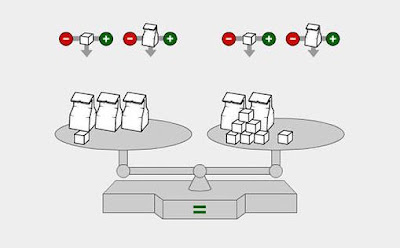## Monday, August 01, 2016

### Balancing Algebraic EquationsThe metaphor of a pan-balance is often used to illustrate the definition of "equation:"

A declaration that two things have the same value (i.e. A = B).

It's an apt analogy, and a multitude of virtual pan-balances exist online to model solving simple algebraic equations.

The idea is that treating both sides of the pan-balance equally (i.e. doing exactly the same thing to both sides) maintains balance while allowing users to isolate unknown numbers on one side and known numbers on the other, from which the value of the unknown can be easily obtained.

It's a lot of fun to play around with balancing equations using a virtual pan-balance manipulative. In doing so, students develop an instinctive sense of how "=" works, a requisite component of intuitive "number sense" required for successful mathematical study.

After learning to balance simple equations using a virtual balance, students can progress to recording the balancing steps on paper – using algebraic notation – rather than carrying out the steps online. Eventually, they can do the steps mentally, using their working memory in place of pencil and paper.

Voila! Mastery!

Following are sites and apps I recommend for practicing balancing simple equations:

-

Sites

Math Is Fun: Balance When Adding and Subtracting

Hoodah Math: Algebra – Balance Equations

Math Playground: Model Algebra

-

Apps

The Fun Way to Learn Algebra: Hands-On Equations (iOS)

The Fun Way to Learn Algebra: Hands-On Equations (Android)

-----